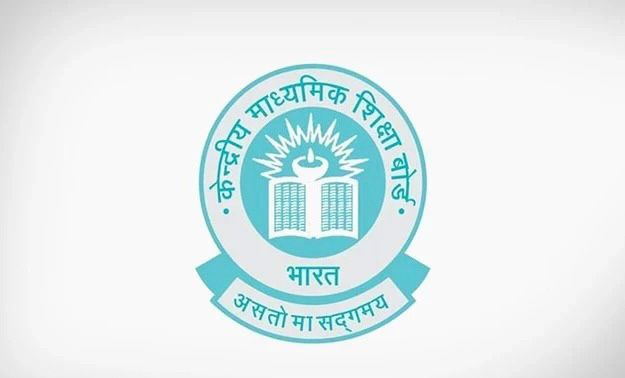# The area of a quadrant of a circle where the circumference of circle is 176 m, is

CBSE Maths Exam 2021-22 Term 1, question: The area of a quadrant of a circle where the circumference of circle is 176 m, isa) 2464 m square

b) 1232 m square

c) 616 m square

d) 308 m square